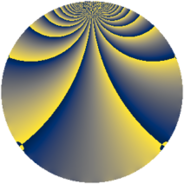# Properties

 Label 760.2.zLevel $760$ Weight $2$ Character orbit 760.z Rep. character $\chi_{760}(349,\cdot)$ Character field $\Q(\zeta_{6})$ Dimension $232$ Newform subspaces $1$ Sturm bound $240$ Trace bound $0$

# Related objects

## Defining parameters

 Level: $$N$$ $$=$$ $$760 = 2^{3} \cdot 5 \cdot 19$$ Weight: $$k$$ $$=$$ $$2$$ Character orbit: $$[\chi]$$ $$=$$ 760.z (of order $$6$$ and degree $$2$$) Character conductor: $$\operatorname{cond}(\chi)$$ $$=$$ $$760$$ Character field: $$\Q(\zeta_{6})$$ Newform subspaces: $$1$$ Sturm bound: $$240$$ Trace bound: $$0$$

## Dimensions

The following table gives the dimensions of various subspaces of $$M_{2}(760, [\chi])$$.

Total New Old
Modular forms 248 248 0
Cusp forms 232 232 0
Eisenstein series 16 16 0

## Trace form

 $$232q - 4q^{4} + 8q^{6} - 112q^{9} + O(q^{10})$$ $$232q - 4q^{4} + 8q^{6} - 112q^{9} + 6q^{10} + 8q^{14} + 10q^{15} - 4q^{16} - 6q^{24} - 2q^{25} - 36q^{26} + 16q^{30} + 16q^{31} - 22q^{34} - 8q^{36} + 8q^{39} - 18q^{40} - 4q^{41} + 42q^{44} - 4q^{46} - 200q^{49} + 28q^{50} + 28q^{54} + 8q^{55} + 48q^{56} - 18q^{60} - 100q^{64} + 12q^{65} + 46q^{66} + 42q^{70} + 52q^{71} + 6q^{76} + 20q^{79} - 34q^{80} - 100q^{81} + 32q^{84} + 20q^{86} - 4q^{89} + 48q^{90} - 116q^{94} - 42q^{95} - 20q^{96} + O(q^{100})$$

## Decomposition of $$S_{2}^{\mathrm{new}}(760, [\chi])$$ into newform subspaces

Label Dim. $$A$$ Field CM Traces $q$-expansion
$$a_2$$ $$a_3$$ $$a_5$$ $$a_7$$
760.2.z.a $$232$$ $$6.069$$ None $$0$$ $$0$$ $$0$$ $$0$$